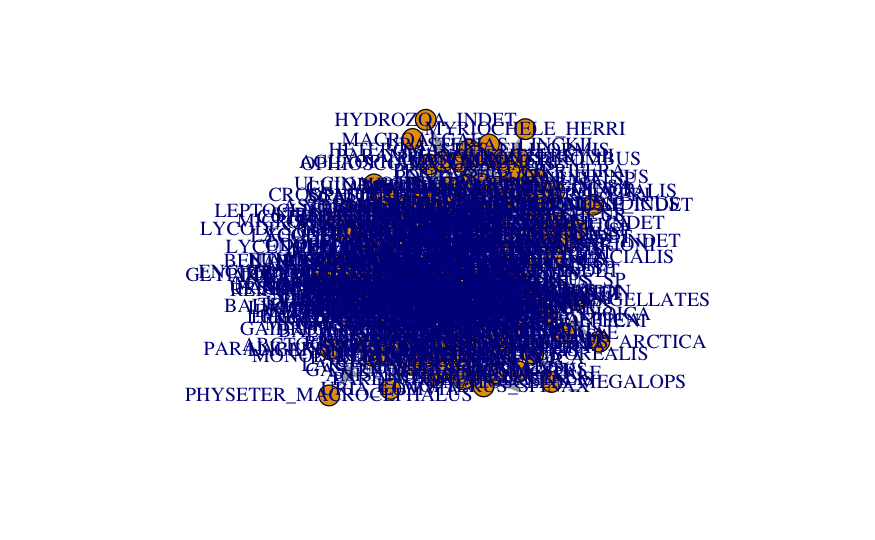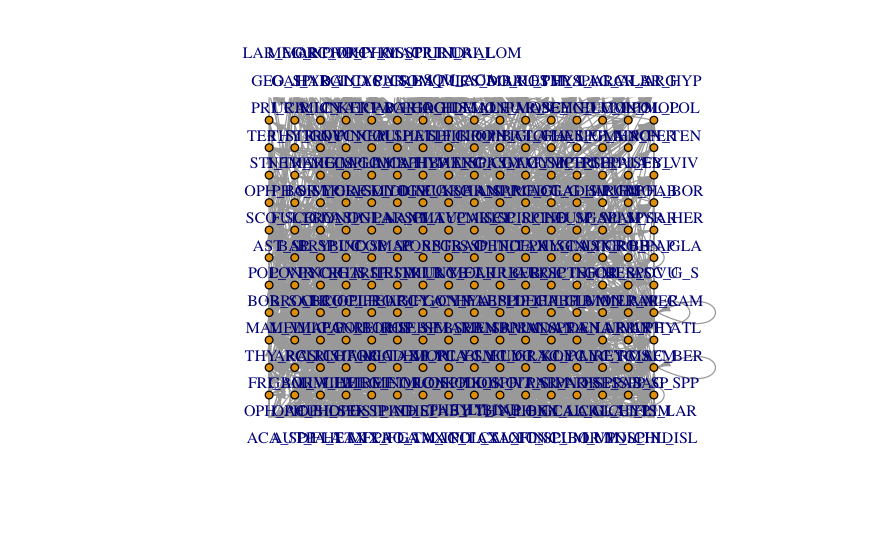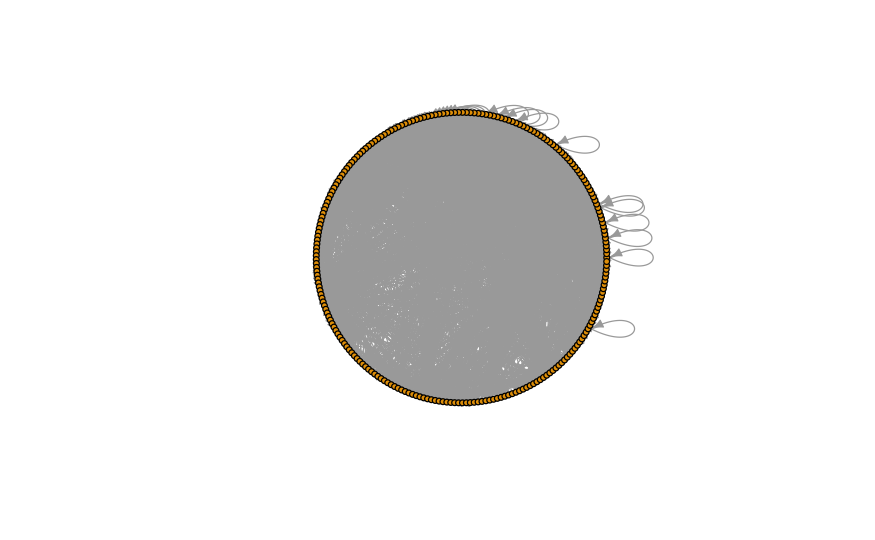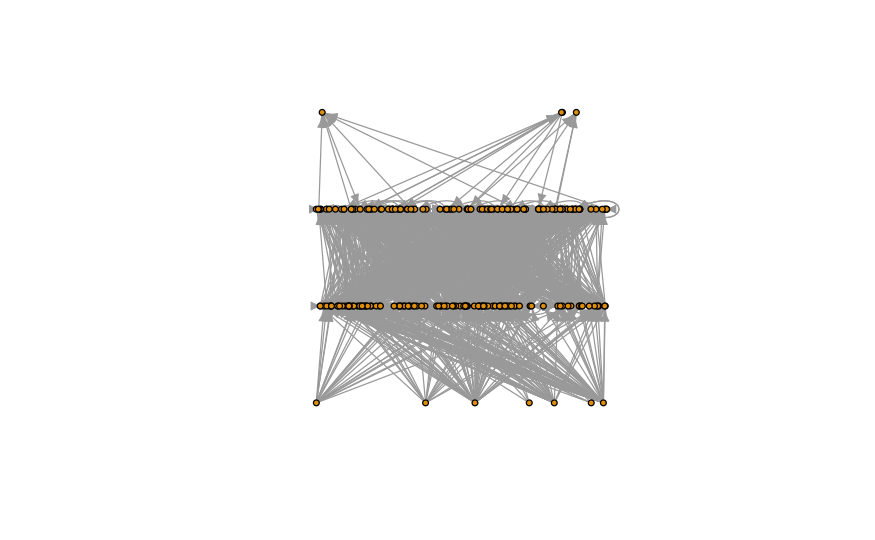# Network Visualization in R (Example) | Drawing Custom Layouts

If you have followed my previous tutorial, you probably noticed that networks (especially large ones) will not display well straight off the bat.

In this tutorial, we will explore layouts for network visualization. Layouts are a way to tell the plot() function how to handle nodes and links in a network graph. It provides coordinates for the nodes and links.

Without the proper layout, nodes and links might end up overlapping because plot() does not know where to place the nodes, if links should be drawn longer to avoid overlaps if there is a hierarchy… and many other elements we might think of when it comes to graphs.

First, if you have not saved the network graph from my last tutorial, run through the tutorial once again to prepare the graph in the igraph format.

The table of content is structured as follows:

## Let’s explore some layouts

First of all, let’s plot our network without any layout to remember how atrocious it can look:

`plot(network_foodweb)`This network is way too big to plot without a layout and some graphical adjustments. Let’s see if controlling the node and label size could help.

```plot(
network_foodweb,
vertex.size = 5, # control node size
vertex.label.cex = 0.8, # control label size (names of the nodes)
vertex.label.dist=1, # control label distance to nodes
edge.arrow.size=.5 # control the size of the arrow
)```A tiny bit better… but way too crowded still.

We can change the labels to make them shorter. The labels represent each species name as in “GENUS_SPECIES”. We will extract the first three letters of each genus and the first three letters of each species. You can check this tutorial or follow here how to do this with the stringr() function.

```vertex_labels <- vertex_attr(network_foodweb, "name") # access vertex attribute "name"
vertex_labels_parts <- vertex_labels |>
stringr::str_split_fixed("_", n = 2)

colnames(vertex_labels_parts) <- c("GENUS", "SPECIES")
vertex_labels_parts <- as.data.frame(vertex_labels_parts)

genus_3let <- stringr::str_sub(vertex_labels_parts\$GENUS, start = 1L, end = 3L)
species_3let <- stringr::str_sub(vertex_labels_parts\$SPECIES, start = 1L, end = 3L)```

Some of the names are not Genus_Species and only contain one string, we need to add something to replace that missing right part.

`species_3let <- ifelse(nchar(species_3let) == 0, "TAX", species_3let)`

Let’s concatenate the parts together

```short_labels <- stringr::str_c(genus_3let, species_3let, sep = "_")
#  "ACA_SPP" "AUT_FLA" "DIA_TAX" "HET_FLA" "MIX_FLA" "PRO_TAX" "GAM_IND" "MAC_TAX" "POL_TAX" "CAL_FIN"```

Let’s set the short labels as a new attribute of the nodes. Make sure they stay in the same order as the names.

```network_foodweb <- set.vertex.attribute(network_foodweb, "short_label", value = short_labels) # set (new) attributes for the nodes
get.vertex.attribute(network_foodweb) # shows the attributes of the nodes```

Let’s plot with the new labels.

```plot(
network_foodweb,
vertex.size = 4, # control node size
vertex.label = V(network_foodweb)\$short_label,
vertex.label.cex = 0.8, # control label size (names of the nodes)
vertex.label.dist=1.2, # control label distance to nodes
edge.arrow.size=.5 # control the size of the arrow
)```Getting there… getting there…

Though controlling of graphic elements is definitely needed, it is insufficient with large complex networks.
When nodes have some tight connections, they tend to clump together.

## Solving everything with a layout

Despite our adjustments, we need a layout to specify nodes coordinates and tell plot() how to deal with overlaps.
A layout is essentially a matrix with two columns containing x and y coordinates for each node that plot() will use to resolve many of our issues.

Over the years, researchers have developed many algorithms that allow to implement various layouts.
The most common have been implemented in R:

```?layout_as_star()
?layout_as_tree()
?layout_in_circle()
?layout_nicely()
?layout_on_grid()
?layout_on_sphere()
?layout_randomly()
?layout_with_dh()
?layout_with_fr()```

Let’s try a random layout to see how it would look:

```l_rand <- layout_randomly(network_foodweb) # compute layout
plot(
network_foodweb,
layout = l_rand, # set layout
vertex.size = 4, # control node size
vertex.label = V(network_foodweb)\$short_label,
vertex.label.cex = 0.8, # control label size (names of the nodes)
vertex.label.dist=1.2, # control label distance to nodes
edge.arrow.size=.5 # control the size of the arrow
)```The random layout stretched out the network and separate some of the nodes clumped in the center. That’s a tiny bit better.

Let’s try the grid layout:

```l_grid <- layout_on_grid(network_foodweb)
plot(
network_foodweb,
layout = l_grid,
vertex.size = 4, # control node size
vertex.label = V(network_foodweb)\$short_label,
vertex.label.cex = 0.8, # control label size (names of the nodes)
vertex.label.dist=1.2, # control label distance to nodes
edge.arrow.size=.5, # control the size of the arrow,
ylim = c(-0.75, .75),
xlim = c(-1, 1)
)```In this graph, I added ylim and xlim. Those two parameters let you recenter the plot area. Visualizations with igraph tend to plot from (0,0), so you can play around with ylim and xlim.

The grid layout arranges nodes at equidistance but might not be suitable for complex graphs and graphs that may contain a hierarchy.

We can try the circle layout before designing our very own layout:

```l_circle <- layout_in_circle(network_foodweb)
plot(
network_foodweb,
layout = l_circle,
vertex.size = 4, # control node size
vertex.label = NA, # NA removes labels and override any label modifiers
vertex.label.cex = 0.8, # control label size (names of the nodes)
vertex.label.dist=0, # control label distance to nodes
edge.arrow.size=.5, # control the size of the arrow,
ylim = c(-1, 1),
xlim = c(-1, 1)
)```As you can see, none of these layouts are satisfactory for such a complex network. It is time to design our very own layout.

## igraph and custom layouts

The food web network contains a natural vertical hierarchy: the food chain where herbivores feed on plants, carnivores eat herbivores, etc.

We can use that vertical hierarchy to split the nodes across levels. Plants belong to the first level, and we will use them as our reference.

We can use a concept called degrees to calculate the number of links in-going and out-going from each node. Plants will have 0 in-going links because they do not feed on anything.

```degree_nodes <- degree(
network_foodweb,
v = V(network_foodweb),
mode = "in"
)
plants_degree0 <- names(degree_nodes[which(degree_nodes == 0)])```

We can use the shortest path, see Network analysis in R to calculate the height of any species in the food chain (i.e., their position).

```distance_to_plant <- distances( # all shortest paths
network_foodweb,
v = V(network_foodweb)[V(network_foodweb)\$name %in% plants_degree0], # select nodes based on their names
to = V(network_foodweb),
mode = "all"
)

shortest_distance_to_plant <- t(distance_to_plant) |>
as_tibble(rownames = NA)  |>
rownames_to_column(var = "species")

shortest_distance_to_plant <- shortest_distance_to_plant |>
rowwise()  |>
mutate(shortest = min(across(plants_degree0)))  |>
identity()

vect_shortest_distance_to_plant <- shortest_distance_to_plant |>
pull(shortest, name = species)
length_to_plant <- vect_shortest_distance_to_plant + 1 # by convention starting at 1```

We got the shortest path to each plant. We can set it as an attribute to the vertices. We will generate a random layout and change the y-axis coordinates to the length we calculate.

```network_foodweb <- set.vertex.attribute(network_foodweb, "position", value = length_to_plant) # set new attributes for the nodes

l_position <- layout_randomly(network_foodweb)
l_position[, 2] <- length_to_plant```

We can plot the final graph.

```plot(
network_foodweb,
layout = l_position,
vertex.size = 4, # control node size
vertex.label = NA, # NA removes labels and override any label modifiers
vertex.label.cex = 0.8, # control label size (names of the nodes)
vertex.label.dist=0, # control label distance to nodes
edge.arrow.size=.5 # control the size of the arrow,

)```The next step would be to adjust the position on the x-axis to spread out the nodes at each level on the y-axis. I am pretty sure you will be able to figure that out on your own following the previous steps.

If you run into trouble, most likely, you have a typo somewhere in your code. If you make a typo, it won’t necessarily throw an error at you because plot.igraph() is a wrapper around the base plot() function. It simply passes arguments to plot().

If the argument does not exist in plot(), then it will just disappear into the void. If you notice the graph did not change upon adding or modifying an argument, check for typos.

Happy network visualization!

## Video, Further Resources & Summary

Do you need more explanations on how to visualize networks using custom layouts? Then you should have a look at the following YouTube video of the Statistics Globe YouTube channel.

Furthermore, you could have a look at some of the other tutorials on Statistics Globe:

This post has shown how to use custom layouts to visualize networks. In case you have further questions, you may leave a comment below.

This page was created in collaboration with Pierre Olivier. You may have a look at Pierre’s author page to read more about his academic background and the other articles he has written for Statistics Globe.

Subscribe to the Statistics Globe Newsletter